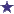# Dispensing Word Problems, including Weight Based Dosing QuestionWeight Based Dosing for Four Divided Doses

1) If the dose of a medication is 30mg/kg/day in four divided doses; what is each dose for a 205 pound patient. ( use 2.2lb/kg and note there are 4 doses per day.)

2) If Rx reads: Cefaclor 250mg/5ml, dispense 150 ml, 375 mg bid(twice daily) x10d (days).

### Comments for Dispensing Word Problems, including Weight Based Dosing Question

Average RatingRating2795mg/day=4 divided doses of 698.75 by: David Question 1) We are given 30mg/kg/day and 205 pounds. First, we will want to calculate the patient's weight in Kilograms. 205 divided by 2.2 = 93.18 Then, multiply 93.18 by 30 = 2795mg/day Next, we need to figure in that there are 4 divided doses from this 2795mg/day. 2795 divided by 4 = 698.75 Question 2) Cefaclor 250/5ml, dispense 150ml, 375mg bid (twice daily) x10d (days) 7.5ml (375mg) BID (twice daily) x10 (for 10 days) This Rx works out to needing exactly 150ml for the total course of therapy.